# Understanding Quadrilaterals Class 8 Notes- Chapter 3

## CBSE Class 8 Maths Chapter 3 Understanding Quadrilaterals Notes:-

To access the complete solutions for class 8 Maths chapter 3 understanding quadrilaterals, click on the below link.

## Introduction to Class 8 Understanding Quadrilaterals

In class 8, the chapter “Understanding Quadrilaterals”, will discuss the fundamental concepts related to quadrilaterals, different types of quadrilaterals and their properties, different types of curves, polygons and some of the theorems related to quadrilaterals such as angle sum property of quadrilaterals, and so on, with complete explanation.

Quadrilaterals are one type of polygon which has four sides and four vertices and four angles along with 2 diagonals. There are various types of quadrilaterals.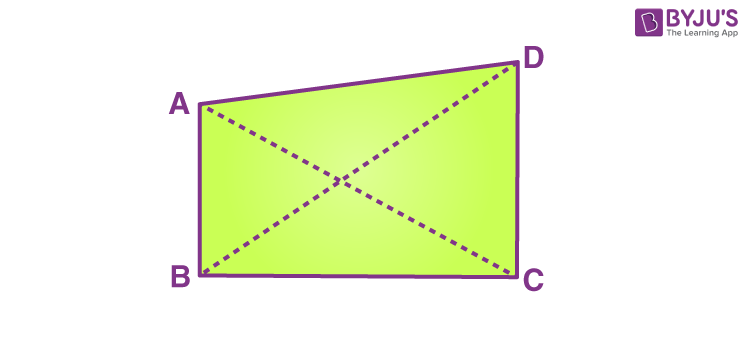The classification of quadrilaterals are dependent on the nature of sides or angles of a quadrilateral and they are as follows:

• Trapezium
• Kite
• Parallelogram
• Square
• Rectangle
• Rhombus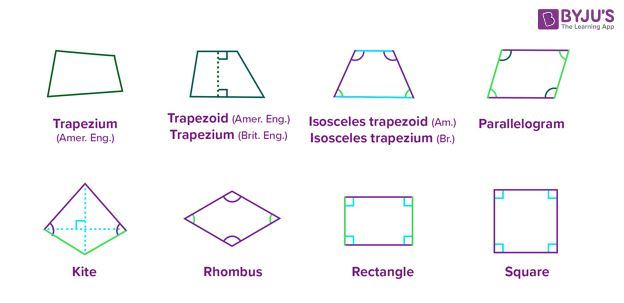The figure given below represents the properties of different quadrilaterals.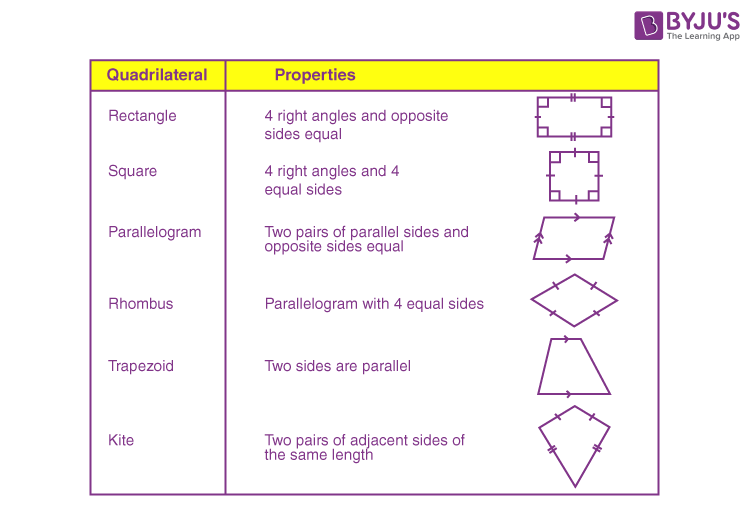## Revisiting Geometry

As we know, Geometry is one of the branches of Mathematics, that deals with the study of different types of shapes, their properties, and how to construct lines, angles and different polygons. Geometry is broadly classified into plane geometry(two-dimensional) and solid geometry (three-dimensional geometry).### Introduction to Curves

A curve is a geometrical figure obtained when a number of points are joined without lifting the pencil from the paper and without retracing any portion. It is basically a line which need not be straight.

The various types of curves are:

• Open curve: An open curve is a curve in which there is no path from any of its point to the same point.
• Closed curve: A closed curve is a curve that forms a path from any of its point to the same point.

A curve can be :

• A closed curve: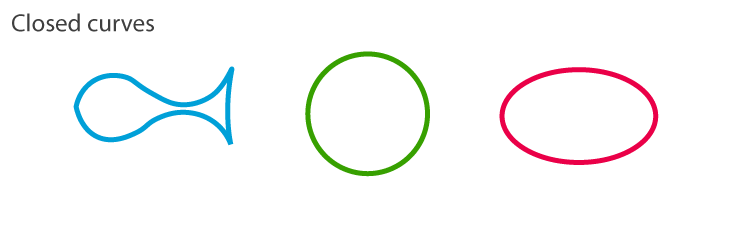•  An open curve: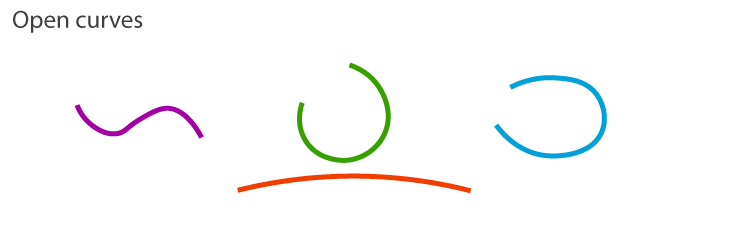• Simple open and closed curves: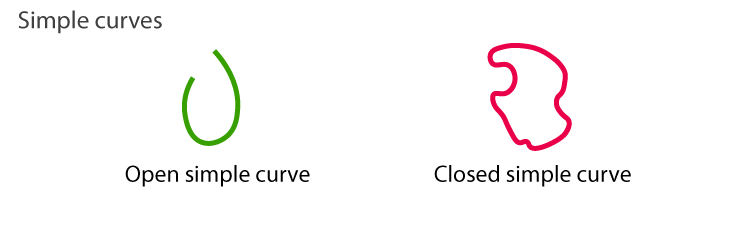To know more about Curve, visit here.

### Polygons

A simple closed curve made up of only line segments is called a polygon.
Various examples of polygons are Squares, Rectangles, Pentagons etc.
Note:
The sides of a polygon do not cross each other.

### Classification of Polygons on the Basis of Number of Sides / Vertices

Polygons are classified according to the number of sides they have. The following lists the different types of polygons based on the number of sides they have:

• When there are three sides, it is triangle
• When there are four sides, it is quadrilateral
• When there are fives sides, it is pentagon
• When there are six sides, it is hexagon
• When there are seven sides, it is heptagon
• When there are  eight sides, it is octagon
• When there are nine sides, it is nonagon
• When there are ten sides, it is decagon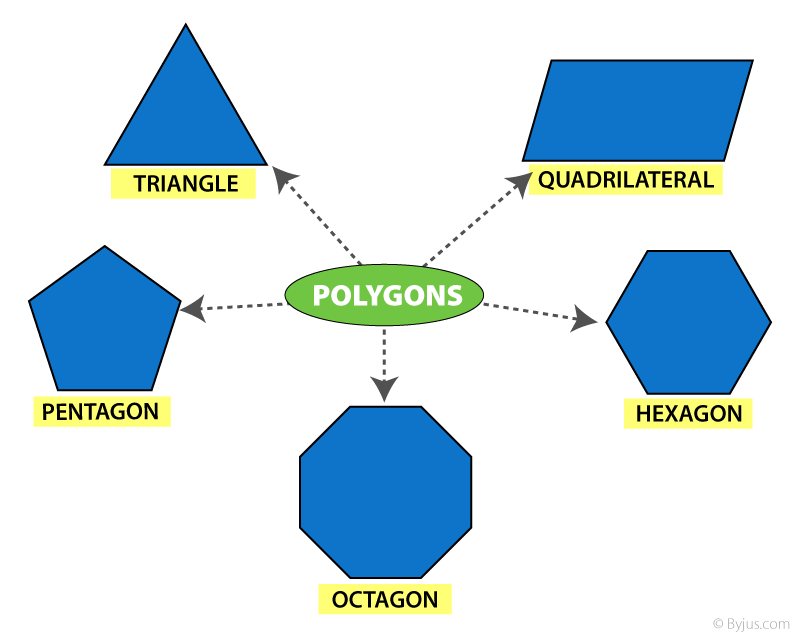To know more about Polygons and its Different Types, visit here.

### Diagonals

A diagonal is a line segment connecting two non-consecutive vertices of a polygon.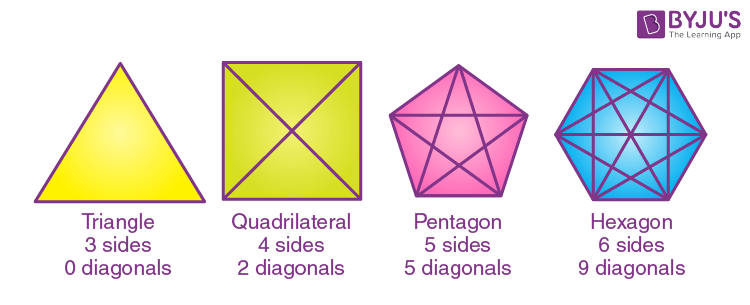### Polygons on the Basis of Shape

Polygons can be classified as concave or convex based on their shape.

• A concave polygon is a polygon in which at least one of its interior angles is greater than 90. Polygons that are concave have at least some portions of their diagonals in their exterior.
• A convex polygon is a polygon with all its interior angle less than 180. Polygons that are convex have no portions of their diagonals in their exterior.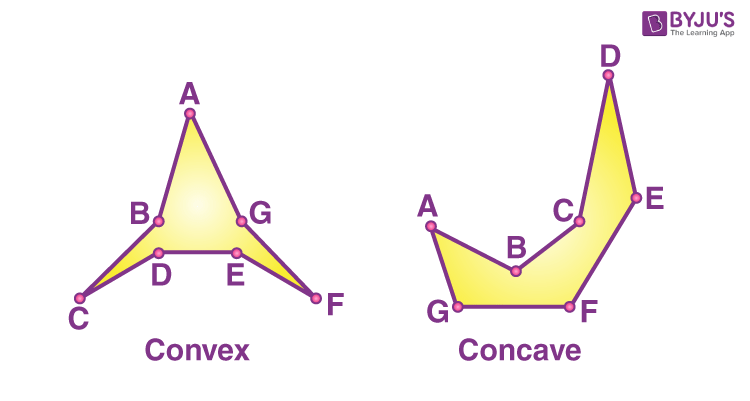To know more about convex and concave polygons, click on the below links:

### Polygons on the Basis of Regularity

Polygons can also be classified as regular polygons and irregular polygons on the basis of regularity.

• When a polygon is both equilateral and equiangular it is called as a regular polygon. In a regular polygon, all the sides and all the angles are equal. Example: Square
• A polygon which is not regular i.e. it is not equilateral and equiangular, is an irregular polygon. Example: Rectangle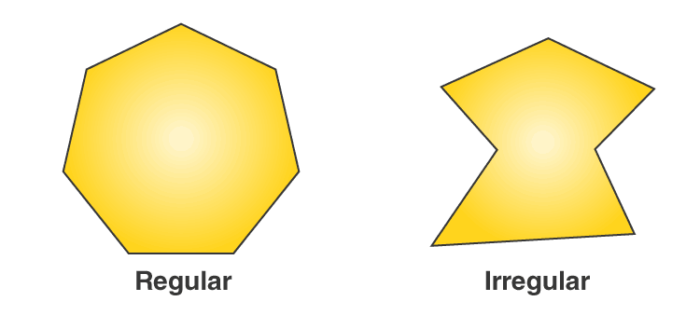### Angle Sum Property of a Polygon

According to the angle sum property of a polygon, the sum of all the interior angles of a polygon is equal to (n2)×180, where is the number of sides of the polygon.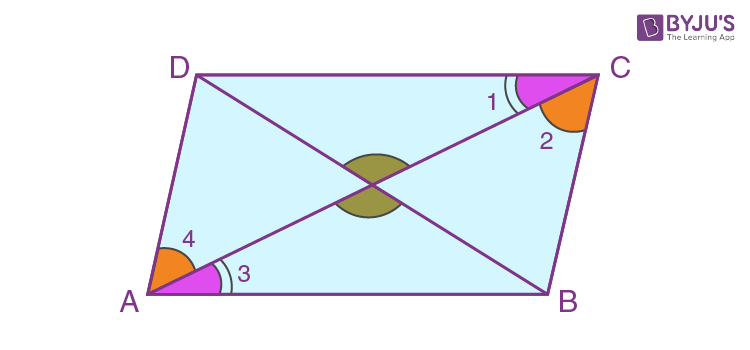As we can see for the above quadrilateral, if we join one of the diagonals of the quadrilateral, we get two triangles.
The sum of all the interior angles of the two triangles is equal to the sum of all the interior angles of the quadrilateral, which is equal to 360 = (42)×180.
So, if there is a polygon which has sides, we can make (n – 2) non-overlapping triangles which will perfectly cover that polygon.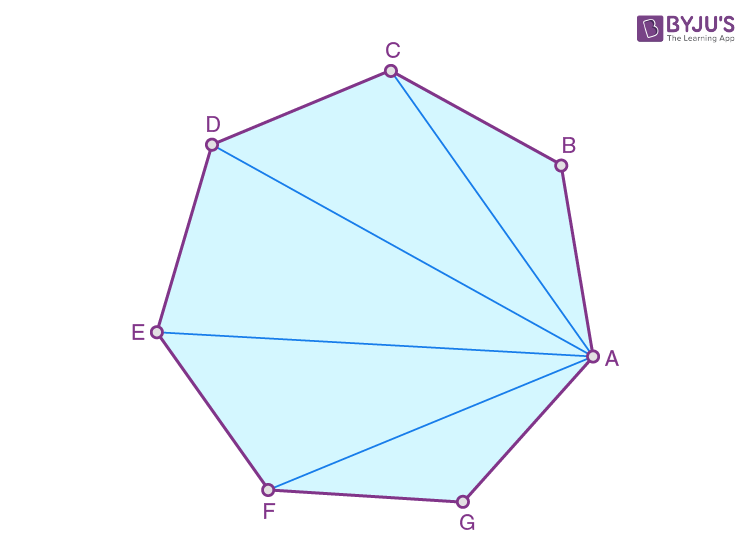The sum of the interior angles of the polygon will be equal to the sum of the interior angles of the triangles = (n2)×180

## To know More About Angle Sum Property of Triangle, Watch the Below Video### Sum of Measures of Exterior Angles of a Polygon

The sum of the measures of the external angles of any polygon is 360.

## Properties of Parallelograms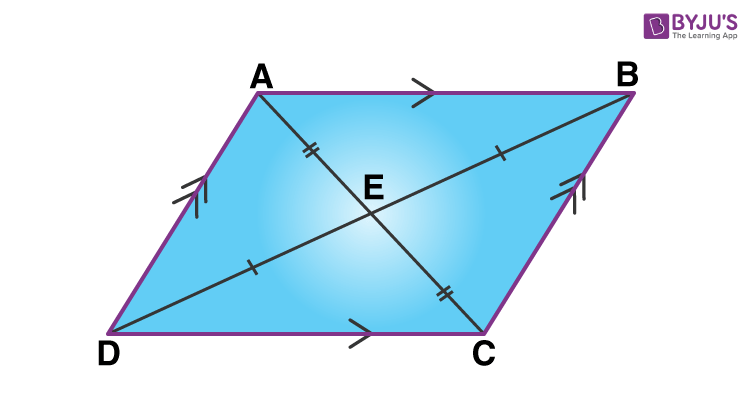The following are the important properties of parallelogram:

1. The opposite sides of a parallelogram are equal and congruent.
2. Diagonals of a parallelogram bisect each other.
3. The diagonals of parallelogram bisect each other and produce two congruent triangles
4. The opposite angles of a parallelogram are congruent.

#### For More Information On Properties Of Parallelogram, Watch The Below Video.### Elements of a Parallelogram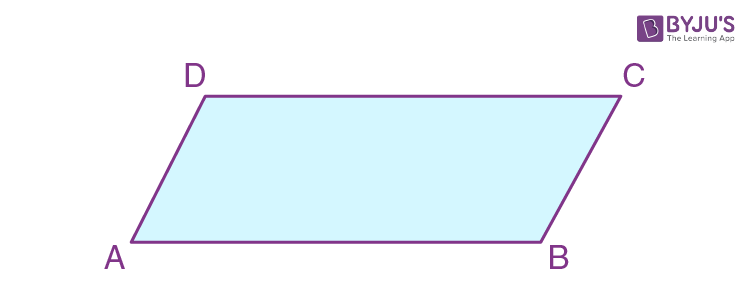• There are four sides and four angles in a parallelogram.
• The opposite sides and opposite angles of a parallelogram are equal.
• In the parallelogram ABCD, the sides ¯¯¯¯¯¯¯¯AB and ¯¯¯¯¯¯¯¯¯CD are opposite sides and the sides ¯¯¯¯¯¯¯¯AB and ¯¯¯¯¯¯¯¯BC are adjacent sides.
• Similarly, ABC and ADC are opposite angles and  ABC and BCD are adjacent angles.

### Angles of a Parallelogram

The opposite angles of a parallelogram are equal.
In the parallelogram ABCD, ABC=ADC and DAB=BCD.

The adjacent angles in a parallelogram are supplementary.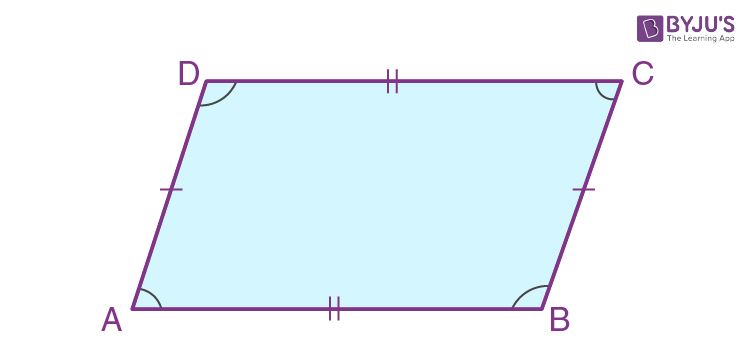For example,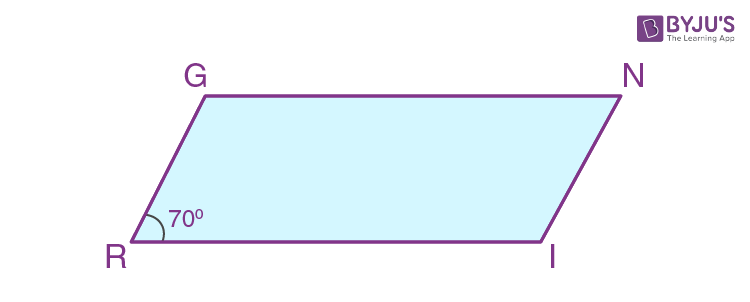In the given parallelogram (RING), ∠R = 70°. Now, we have to find the remaining angles.

As we know, the opposite angles of a parallelogram are equal, we can write:

∠R = ∠N = 70°.

And we know, the adjacent angles of a parallelogram are supplementary, we get

∠R + ∠I = 180°

Hence, ∠I = 180° – 70° = 110°

Therefore, ∠I = ∠G = 110° [Since ∠I and ∠G are opposite angles]

Hence the angles of a parallelogram are ∠R = ∠N = 70° and ∠I = ∠G = 110°.

### Diagonals of a Parallelogram

The diagonals of a parallelogram bisect each other at the point of intersection.
In the parallelogram ABCD given below, OA = OC and OB = OD.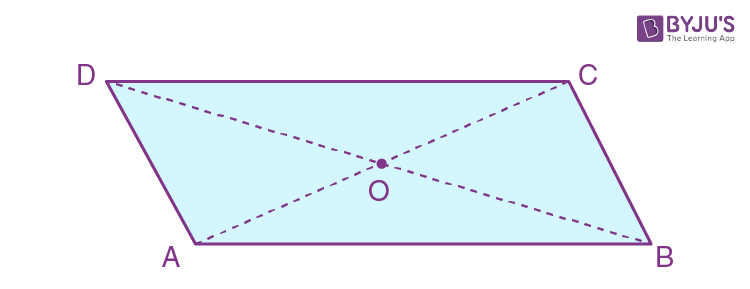Consider an example, if OE = 4cm and HL is five more than PE, find the measure of OH.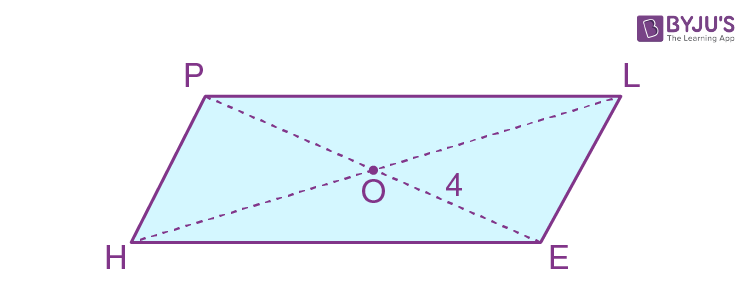Given that, OE = 4 cm and hence, OP = 4cm [Since OE = OP]

Hence PE =OE + OP = 4cm + 4cm = 8 cm

Also given that, HL is 5 more than PE,

Hence, HL = 5 + 8 = 13 cm.

Therefore, OH = HL/2 = 13/2 = 6.5 cm

Therefore, the measurement of OH is 6.5 cm

## Properties of Special Parallelograms

### Rectangle

A rectangle is a parallelogram with equal angles and each angle is equal to 90.
Properties:

• Opposite sides of a rectangle are parallel and equal.
• The length of diagonals of a rectangle is equal.
• All the interior angles of a rectangle are equal to 90.
• The diagonals of a rectangle bisect each other at the point of intersection.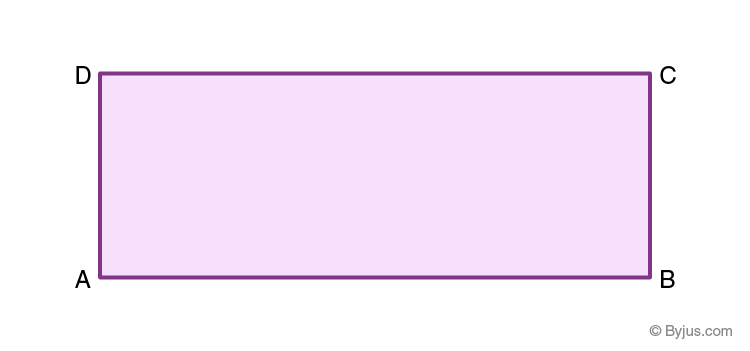### Square

A square is a rectangle with equal sides. All the properties of a rectangle are also true for a square.
In a square the diagonals:

• bisect one another
• are of equal length
• are perpendicular to one another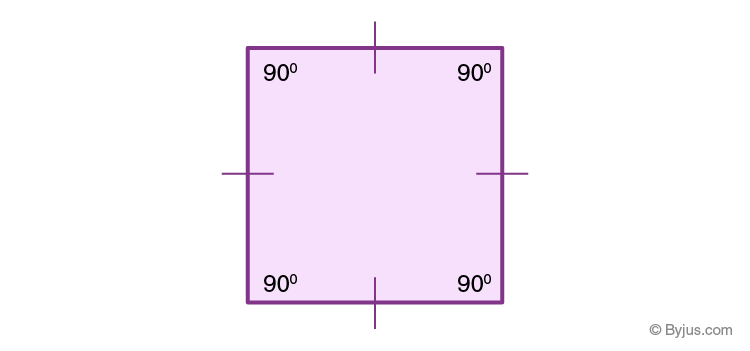## Rhombus

Rhombus is one of the special cases of parallelogram. In Rhombus, all the sides are equal and the opposite sides are also equal.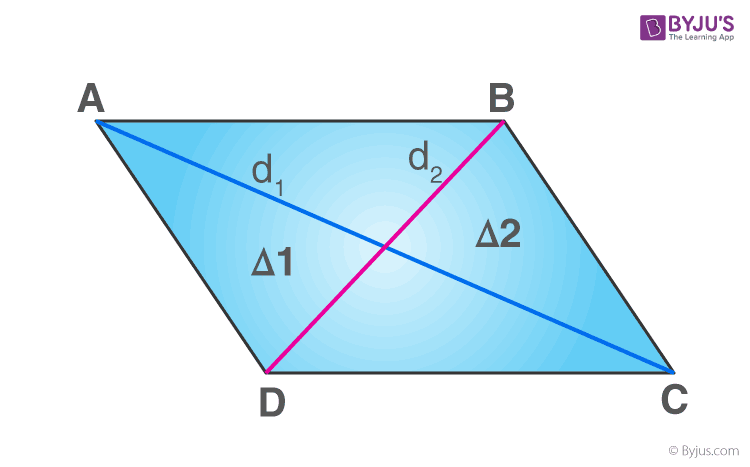## Frequently asked Questions on CBSE Class 8 Maths Notes Chapter 3: Understanding Quadrilaterals

### What is a ‘Curve’?

A curve refers to a line that is not straight. In other words, it may be any line that is bent to some extent.

### What is a ‘convex polygon’?

Convex polygon is a polygon each of whose angles is less than a straight angle.

### What are the properties of a ‘Parallelogram’?

1. Opposite sides are congruent 2. Opposite angels are congruent 3. Consecutive angles are supplementary. 4. Daigonals of a parallelogram bisect